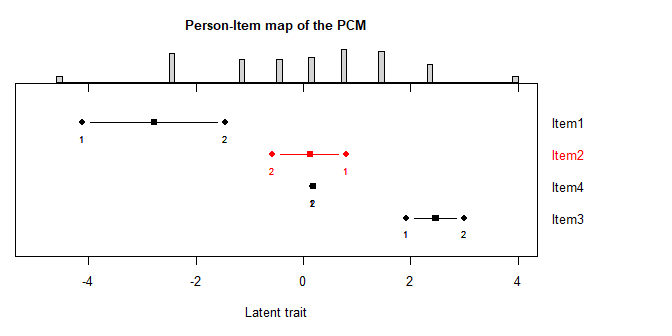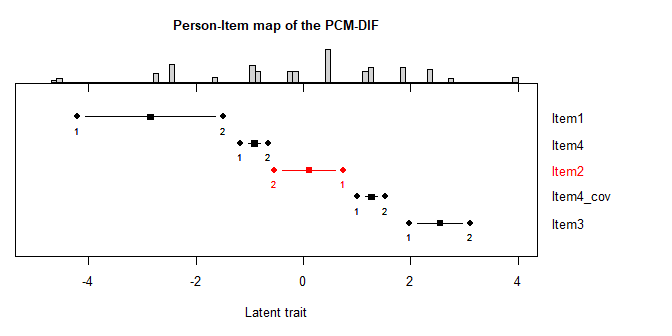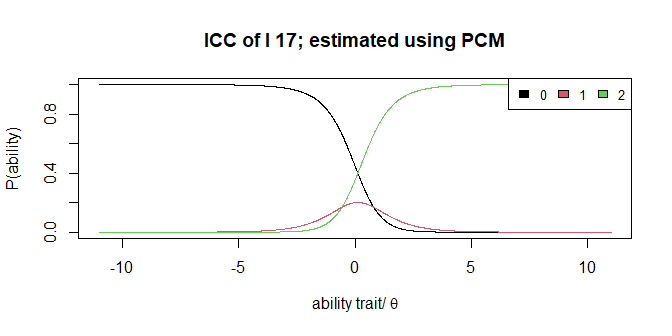# autoRasch: Working with the models

# install.packages("remotes")
remotes::install_github("fwijayanto/autoRasch", build_manual = TRUE, build_vignettes = TRUE)
library(autoRasch)

In this autoRasch package four models are implemented, i.e., the PCM, the GPCM, the PCM-DIF, and the GPCM-DIF. For binary responses, the PCM and GPCM will transform to the Rasch model and the 2-PL model, respectively.

### The PCM and PCM-DIF

The Partial Credit Model (PCM) is the model multi-categorical responses, which generalizes the Rasch model.

pcm_res <- pcm(shortDIF)

Similar with the PCM the PCM-DIF generalize the Rasch model even more by parameterizing the DIF effect in the items. However, in the PCM-DIF, we need to define the groups of the subjects. In this simulated dataset, the groups are pre-designed as the first half and the rest.

grMap <- matrix(c(rep(0,50),rep(1,50)),ncol = 1, dimnames = list(c(1:100),c("cov")))
pcmdif_res <- pcm_dif(shortDIF, groups_map = grMap)

Some of the S3 generic functions are implemented to this model, i.e., summary() and print(). However, to filter which model’s parameter to plot, I add par argument. It could be filled in using theta, beta, gamma, alpha, and delta, depends on the models applied. Ignoring this argument shows all estimated parameters of the model. Item Loc. represents the item location which is obtained by averaging the threshold values. * indicates the occurence of a disordered threshold.

summary(pcm_res, par="beta")
#>
#>
#> The estimated difficulty scores:
#>        Th_1  Th_2 Item Loc.
#> Item1 -4.12 -1.46     -2.79
#> Item2  0.80 -0.57      0.12 *
#> Item3  1.92  3.00      2.46
#> Item4  0.16  0.19      0.17
#>
#> The most difficult item:  Item3
#> The easiest item:  Item1
#> There are 1 items which have disordered thresholds.
#> '*' Item has disordered thresholds.
summary(pcmdif_res, par="delta")
#>
#>
#> The estimated DIF effects (gap of difficulties) (delta):
#>        cov
#> Item4 2.18

For PCM and PCM-DIF, the estimated parameters could be plotted as a Person-Item map. However, as for PCM-DIF the items with DIF will be resolved by splitting the items based on the given groups. As the results, we may obtain more than one item with the same number.

plot_PImap(pcm_res, main = "Person-Item map of the PCM")plot_PImap(pcmdif_res, main = "Person-Item map of the PCM-DIF")Since item19 is a DIF-item, there are two item19 in the Person-Item map of the PCM-DIF, the I 19 and I 19_a for the reference and the focal group, respectively. Red color means that there is a threshold disorder within the item.

The plot_ICC() and plot_EVC() could be used to plot the item characteristic curve (ICC) and the expected value curve (EVC), respectively, for each item.

plot_ICC(pcm_res, itemno = 2, main = "ICC of I 17; estimated using PCM")To implement the standard Rasch goodness-of-fit statistics, a specific S3 function have been developed to compute the (item/person) fit statistics, i.e., fitStats() function, for the PCM and the PCM-DIF. To summarize only item or person statistic, itemfit() or personfit() could be used, respectively.

pcm_fit <- fitStats(pcm_res)
itemfit(pcm_fit)
#>
#> Item Fit Statistics:
#>
#>       OutfitMnSq InfitMnSq OutfitZSTD InfitZSTD Alpha
#> Item1       0.40      0.57      -1.86     -2.78  1.16
#> Item2       0.41      0.66      -1.61     -2.59  1.14
#> Item3       0.57      0.71      -0.66     -1.60  1.09
#> Item4       0.96      1.16      -0.02      1.14  0.93
pcmdif_fit <- fitStats(pcmdif_res)
itemfit(pcmdif_fit)
#>
#> Item Fit Statistics:
#>
#>       OutfitMnSq InfitMnSq OutfitZSTD InfitZSTD Alpha
#> Item1       0.32      0.49      -2.12     -3.48  1.18
#> Item2       0.30      0.47      -2.08     -4.30  1.18
#> Item3       0.48      0.62      -0.81     -2.29  1.10
#> Item4       0.51      0.71      -1.36     -2.04  1.11

### The GPCM and GPCM-DIF

The Generalized Partial Credit Model (GPCM) generalizes the Partial Credit Model by modelling the predictability of the subjects’ responses (discrimination parameters).

gpcm_res <- gpcm(shortDIF)

For further generalization to the PCM, the Generalized Partial Credit Model with DIF (GPCM-DIF) not only models the predictability, but also the effects of the differential functioning of each items.

grMap <- matrix(c(rep(0,50),rep(1,50)),ncol = 1, dimnames = list(c(1:100),c("cov")))
gpcmdif_res <- gpcm_dif(shortDIF, groups_map = grMap)

Similar to the PCM and PCM-DIF, some of the S3 generic functions such as summary() and print() also applied to these models.

summary(gpcm_res, par="alpha")
#>
#>
#> The estimated discrimination parameters:
#>           alpha
#> Item1 1.1603282
#> Item2 1.1403392
#> Item3 1.0904078
#> Item4 0.9339005
summary(gpcmdif_res, par="delta")
#>
#>
#> The estimated DIF effects (gap of difficulties):
#>           cov
#> Item4 2.23808

Unlike plot_PImap() which only implemented to the PCM and PCM-DIF, plot_EVC() and plot_ICC() are also implemented to the GPCM and the GPCM-DIF.